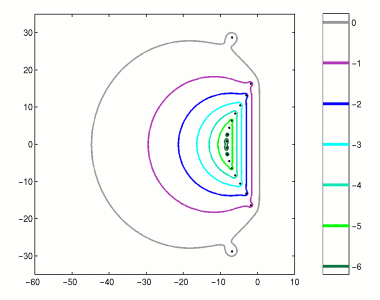Home | Introduction | Software | Examples | History | People | Bibliography | Applications | Theorems

## Pseudospectra of a Spectral Approximation of the First Derivative Dimension N=17This figure illustrates approximate pseudospectra of the first derivative operator d/dx on [-1,1] with boundary condition u(1)=0. The pseudospectra of the infinite dimensional operator consist of half-planes. This is suggested in this low-dimensional discretization by the straight lines that form the rightmost part of each pseudospectral boundary. For more information, see [Tre92].

Use the following MATLAB code compute a similar image using EigTool.

```  [D,x] = cheb(18);       % cheb.m from Trefethen's "Spectral Methods in MATLAB"
A = D(2:end,2:end);
opts.npts=50;
opts.ax = [-60 10 -35 35];
opts.levels = -6:0;
eigtool(A,opts)
```